# Reversal Trading with Fractal Wave and Stochastic Cycles

In the previous chapters, we demonstrated the practical application of Geometric Prediction in the financial trading. In doing so, we have shown several different ways to detect geometric regularity. Then, we tried to combine them with turning point probability (i.e. statistical regularity) to fulfill the technical version of Geometric Prediction.

Geometric Prediction (General version) = statistical regularity + geometric regularity + knowledge around object

Geometric Prediction (Technical version) = statistical regularity + geometric regularity

The approaches to detect the geometric regularity include Support, Resistance, Harmonic Pattern, Falling Wedge pattern, Rising Wedge pattern, and Gann Angles. This is not necessarily the exhausted list of the approaches to detect the geometric regularity. With these approaches, our decision making process for buy and sell decision-making included two steps like below.

Step 1: Detect geometric regularity in price series

Step 2: Confirm the geometric regularity with statistical regularity

For example, in the case of using Harmonic Pattern, we detect Harmonic pattern (Step 1). Then we use the turning point probability in price and time to confirm the timing provided from the Harmonic Pattern (Step 2). Likewise, the rest of approaches will follow more or less the similar steps. In this chapter, we will be showing how to use above two steps in the reverse order. Hence, we will be making our trading decision like below:

Step 1: Detect statistical regularity in price series

Step 2: Confirm the statistical regularity with geometric regularity

To demonstrate the two reversed steps, we will use the confluence pattern of mother wave and child wave with high turning point probability (Step 1). Then we will use Fibonacci Expansion to get the fine tune of the possible turning point (Step 2).

The importance of the confluence pattern of mother wave and child wave was discussed heavily in many parts of this book. The confluence price pattern can be used to predict the multiple stochastic cycles present in fractal waves. Anatomy of the confluence price pattern is shown in Figure 4.8-1. By definition, the confluence price pattern is the mother wave pattern made up from several child fractal triangles. Some example of fractal triangles are shown in Figure 4.8-2. In fact, we can have various combination of child fractal triangles to form the mother wave.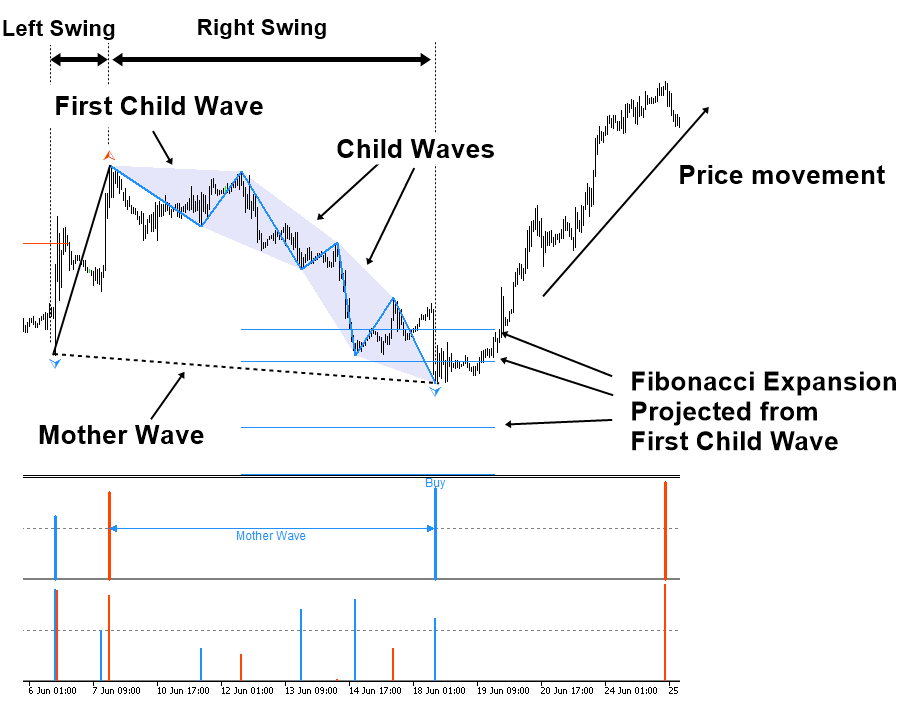Figure 4.8-1: The anatomy of the confluence pattern in EURUSD H1 timeframe

In this book, we detect the confluence price pattern if more than two child fractal triangles present inside the right swing of the mother wave. The confluence price pattern shown in Figure 4.8-1 looks like the cup and handle price pattern. In fact, the confluence pattern sometimes look like AB=CD pattern when there are two child fractal triangles in the right swing of the mother wave. Sometimes, they look like Elliott Wave 12345 pattern when there are three child fractal triangles in the right swing of the mother wave. Sometimes, their turning point can overlap with Harmonic Pattern and X3 price patterns.Figure 4.8-2: Four different fractal triangles in the financial market.

To detect statistical regularity (Step 1), we can consider the amplitude and wavelength of the mother wave at the right swing. It is possible to consider the amplitude and wavelength of the last child wave inside the mother wave too. However, our priority is to catch the big price movement. Hence, the amplitude and wavelength of the mother wave is more important.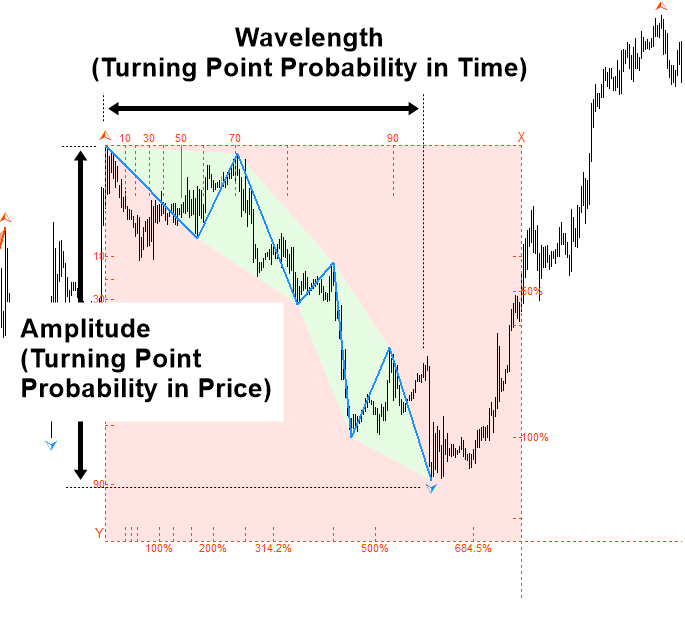Figure 4.8-3: Turning Point Probability for the mother wave

To make use of the statistical regularity, we can detect the sufficiently large amplitude and wavelength at the right swing of the mother wave. For example, it might be sensible to choose amplitude with over 70% or 80% turning point probability to start with. Once such a confluence pattern is detected in chart, then we can use Fibonacci expansion to tune the buy or sell timing. We can draw Fibonacci expansion from the first child fractal triangle (=Y0/Y1) as shown in Figure 4.8-4. For your information, in Figure 4.8-4, we have six child fractal triangles inside the right swing of the mother wave.Figure 4.8-4: Confluence price pattern detected in EURUSD H1 timeframe

For your trading, some sensible Fibonacci expansion ratios are listed in Table 4.8-1. For each confluence pattern, we only need to concern several of these ratios per our situation. The most important factor for choosing the ratio is the size of the first child fractal wave.

 Fibonacci Expansion ratio Fibonacci Expansion ratio in percent 0.382 38.2% 0.618 61.8% 1.000 100.0% 1.618 161.8% 2.000 200.0% 2.618 261.8% 3.148 314.8% 4.236 423.6% 5.000 500.0% 6.845 684.5%

Table 4.8-1: List of possible Fibonacci Expansion ratio for our trading

In Table 4.8-2, we shares some observations we made in the past about these ratios. For example, when the amplitude of the child fractal wave is somewhere between 0% and 20%, we need to focus more on the ratios of 2.618, 3.148, 4.236, 5.000, and 6.845 than other ratios. Likewise, when the amplitude of the child fractal wave is somewhere between 21% and 40%, we need to focus more on the ratios of 1.618, 2.000, 2.618, and 3.148 than other ratios. What we have noticed is that it is slightly easier to trade if the size of first child fractal wave is big. Especially, when the size of child fractal wave is over 50%, we only need to concern two or three Fibonacci expansion ratios to tune our entry. Although the turning point might happen on some other ratios too, the listed ratios in Table 4.8-2 can provide you the rule of thumb for your trading. However, please be aware that Table 4.8-2 is not exhausted list of the ratios to consider.

 Amplitude of First Child Fractal Wave Fibonacci Expansion ratios 0% to 20% 2.618, 3.148, 4.236, 5.000, 6.845 21% to 40% 1.618, 2.000, 2.618, 3.148 41% to 60% 0.618, 1.000, 1.618, 2.000 61% to 80% 0.382, 0.618, 1.000, 1.618 81% to 95% 0.382, 0.618

Table 4.8-2: List of important Fibonacci Expansion ratios per the size of first child fractal wave

So far, we have discussed some essential knowledge to trade with the confluence pattern of mother wave and child wave. Let us go through some practical examples. In Figure 4.8-5, we present the confluence price pattern that are made up from two child fractal triangles in the right swing of the mother wave. We detected this pattern when the right swing of the mother wave was nearly hitting 80% turning point probability. Then we used the Fibonacci expansion for the fine tune of the entry. In fact, the turning point was made at 1.618 ratio. The final turning point probability of the mother wave was 95% (M0) in price and 88% in time. As you can see, the amplitude (C0) of first child fractal wave was around 49% turning point probability. It helps us to anticipate the turning point at the Fibonacci Expansion ratios of 1.000, 1.618, and 2.000.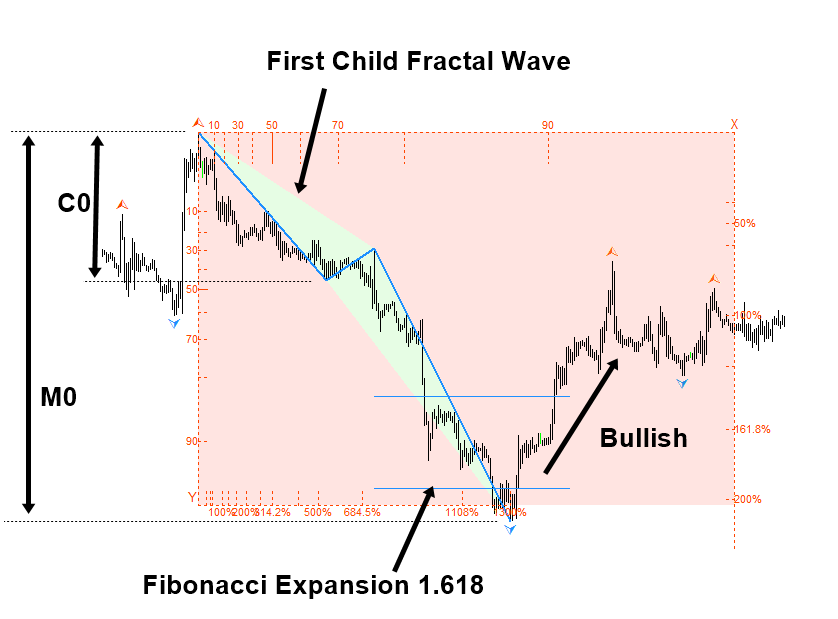Figure 4.8-5: Confluence price pattern with two child fractal triangles inside the right swing of the mother wave in EURUSD H1 timeframe

In Figure 4.8-6, we present another example of the confluence price pattern with eight child fractal waves in the right swing of the mother wave. Initially we detected this price pattern around 80% turning point probability of the mother wave.  Then we used the Fibonacci expansion for the fine tune of the entry. The size of first fractal triangle was around 10%. Hence, we were anticipating bit wider range of Fibonacci expansion ratios from 2.618 to 5.000. The actual turning point was taken at the Fibonacci expansion 3.148 ratio. The final turning point was made around 88% and 95% turning point probability in price and time.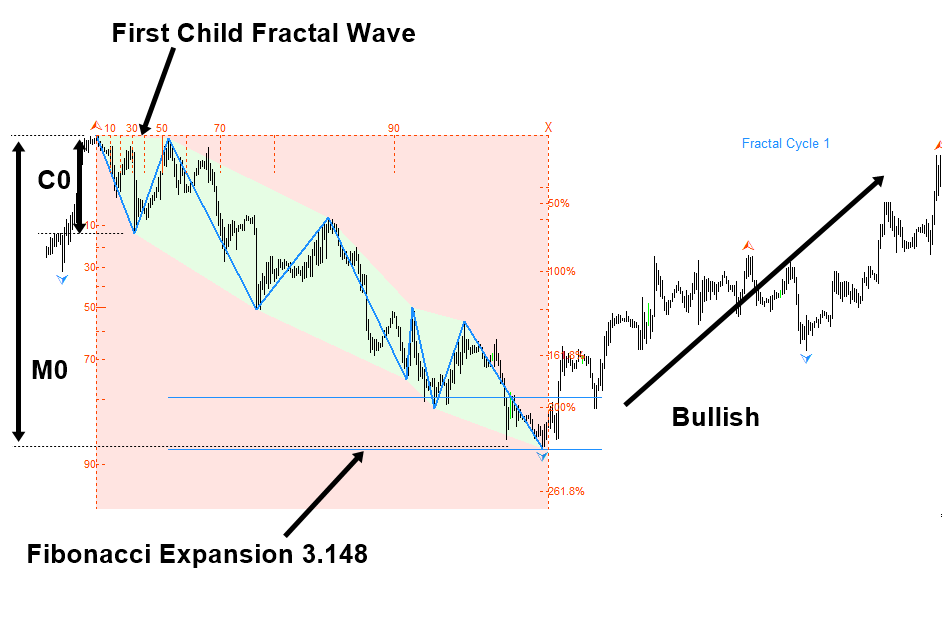Figure 4.8-6: Confluence price pattern with eight child fractal triangles inside the right swing of the mother wave in EURUSD H1 timeframe

Sometimes, you might have the question like “I detected the confluence pattern look like Elliott Wave 12345 pattern. Can I apply Elliott Wave trading with the confluence pattern?”. The answer is “Yes, you can”.  This typically happens when we detect the confluence pattern with three child fractal triangles in the right swing of the mother wave (Figure 4.8-7). Elliott Wave pattern detection is one way of ensuring the geometric regularity like Harmonic Pattern or X3 Price Pattern using the Ratios and Wave principle. Hence, applying Elliott Wave pattern does not go against the trading principle with the Geometric Prediction. In such case, you should apply the two steps we have provided in this chapter first. Then you will use the additional knowledge of Elliott Wave to make your final decision. In any case, the size of first child fractal wave provides an important clue about the possible turning point. Hence, we do not recommend you to skip this process.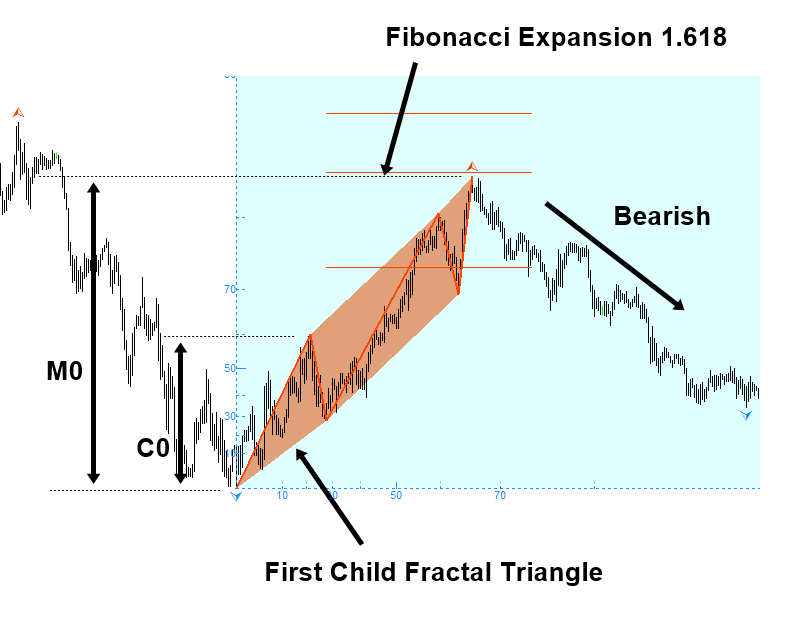Figure 4.8-7: Confluence price pattern with three child fractal triangles look like Elliott Wave 12345 pattern in GBPUSD H1 timeframe

You can have a similar question in the case of Harmonic Pattern too. For example, you can have a harmonic pattern coincided with the turning point of the confluence price pattern. For example, in Figure 4.8-8, the turning point of the confluence price pattern is coincided with the sell signal of Butterfly pattern. As in the Elliott wave pattern, this does not go against our trading principle with the Geometric Prediction. We can just use this additional knowledge with Harmonic Pattern to confirm the geometric regularity. Just make sure that you follow the step 1 and step 2 provided in this chapter first. Then apply your Harmonic Pattern knowledge as an additional confirmation tool. Since several different methods agree on the trading direction, this can improve the trading performance in long run.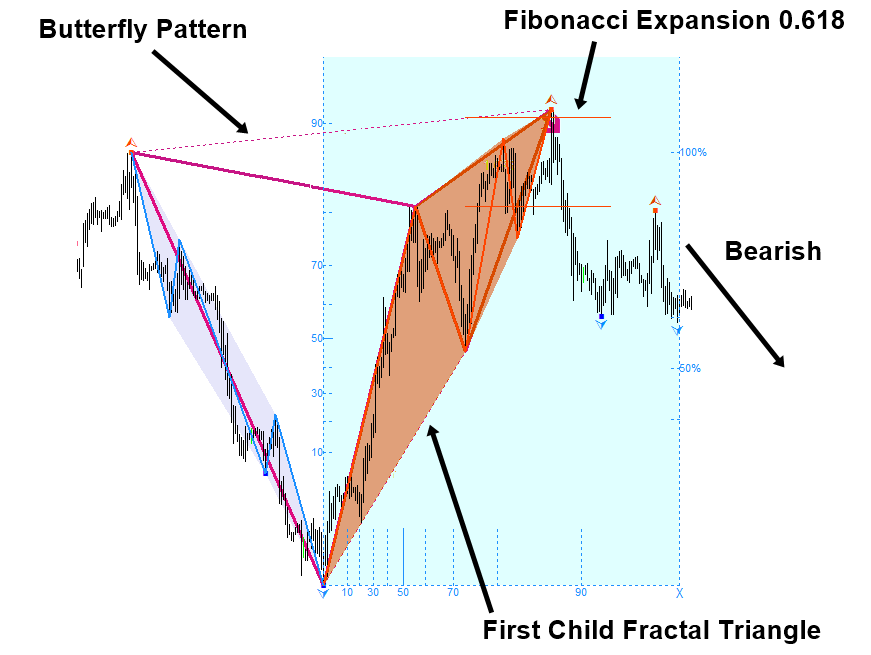Figure 4.8-8: Confluence price pattern with four child fractal triangles, which coincides with Butterfly pattern in GBPUSD H1 timeframe

Likewise, you can apply the similar logic to the case of Support, Resistance, Falling Wedge pattern, and Rising Wedge pattern. You can use all these approaches to extend the ability to detect geometric regularity. Combining the statistical regularity and geometric regularity completes the technical version of the Geometric prediction. To complete the prediction, we also recommend adding your knowledge on the market and fundamental analysis behind the financial instrument (i.e. knowledge around the object) during the decision-making. This would provide the most complete and accurate form of the market prediction fulfilling all the essential parts of the Geometric Prediction.

Below is the landing page for Fractal Pattern Scanner for MetaTrader 4 and MetaTrader 5. The same products are available on www.mql5.com too.

https://www.mql5.com/en/market/product/49170

https://www.mql5.com/en/market/product/49169